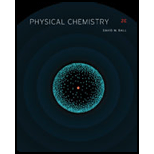# Although first-order diffractions might occur at angles greater than 30 ° , if they do there will not be a second-order (or higher) diffraction. Use Bragg’s law to argue why this is the case. (Consider the properties of the sine function at angles greater that 30 ° .)### Physical Chemistry

2nd Edition
Ball + 3 others
ISBN: 9781133958437

#### Solutions

Chapter
Section### Physical Chemistry

2nd Edition
Ball + 3 others
ISBN: 9781133958437
Chapter 21, Problem 21.24E
Textbook Problem
1 views

## Although first-order diffractions might occur at angles greater than 30 ° , if they do there will not be a second-order (or higher) diffraction. Use Bragg’s law to argue why this is the case. (Consider the properties of the sine function at angles greater that 30 ° .)

Interpretation Introduction

Interpretation:

The reason as to why there would not be second-order diffraction if the first-order diffractions occur at angles greater than 30° is to be explained.

Concept introduction:

A unit cell of the crystal is the three-dimensional arrangement of the atoms present in the crystal. The unit cell is the smallest and simplest unit of the crystal which on repetition forms an entire crystal. The parameters of a crystal can be obtained experimentally by X-ray diffraction technique.

### Explanation of Solution

The Bragg equation for diffraction of X rays is given by an expression as shown below.

nλ=2dsinθ …(1)

Where,

λ is the wavelength of the incident ray.

d is the distance between two parallel planes.

θ is the Bragg’s angle.

n is the order of diffraction.

The value of n for first-order diffractions is 1.

The given Bragg’s angle for first-order diffractions is 30°.

Substitute the value of θ and n in the equation (1).

(1)λ=2dsin30°λ=2d(0.5)λ=d

When Bragg’s angle is greater than 30°, then the above expression will change to λ>d

### Still sussing out bartleby?

Check out a sample textbook solution.

See a sample solution

#### The Solution to Your Study Problems

Bartleby provides explanations to thousands of textbook problems written by our experts, many with advanced degrees!

Get Started

Find more solutions based on key concepts
Determine the mass percentage of carbon in CO and CO2.

Chemistry for Today: General, Organic, and Biochemistry

The National Center for Complementary and Alternative Medicine fNCCAMl promotes laetrile therapy. T F

Nutrition: Concepts and Controversies - Standalone book (MindTap Course List)

What is physically exchanged during crossing over?

Human Heredity: Principles and Issues (MindTap Course List)

________ is lifes primary source of energy. a. Food b. Water c. Sunlight d. ATP

Biology: The Unity and Diversity of Life (MindTap Course List)

A rocket for use in deep space is to be capable of boosting a total load (payload plus rocket frame and engine)...

Physics for Scientists and Engineers, Technology Update (No access codes included)

How can sound be used to measure the temperature of an ocean?

Oceanography: An Invitation To Marine Science, Loose-leaf Versin